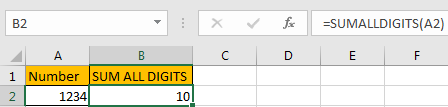# How to Sum All Digits in A Cell in Excel

If we enter a number 1234 in a single cell and we want to get the sum of all digits like 1+2+3+4=10 in another cell, how can we do? As there is no function like SUM to sum all digits in a cell, we need to use a formula with some functions together to do sum.

Precondition:

Can you sum all digits in a number quickly?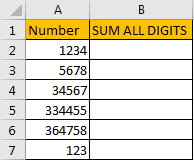## Method 1: Sum All Digits in A Cell by Formula

Step 1: In B1 enter the formula =SUMPRODUCT(1*MID(A2,ROW(INDIRECT(“1:”&LEN(A2))),1)). Then click ENTER.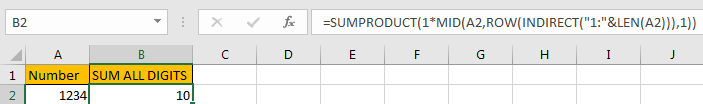Step 2: Drag down the fill handle till reaching the last cell in the table.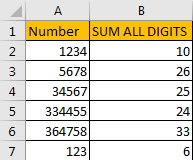## Method 2: Sum All Digits in A Cell by User Defined Function Via VBA Code

This formula is so long and contains many functions, if we can create a function to sum all digits, it will be much better. Actually, we can via VBA code to define a user defined function.

Step 1: On current visible worksheet, right click on sheet name tab to load Sheet management menu. Select View Code, Microsoft Visual Basic for Applications window pops up.

Or you can enter Microsoft Visual Basic for Applications window via Developer->Visual Basic. You can also press Alt + F11 keys simultaneously to open it.

Step 2: In Microsoft Visual Basic for Applications window, click Insert->Module, enter below code in Module1:

```Function SUMALLDIGITS(Num As String) As Integer

Dim Sum As String

Dim i As Integer

For i = 1 To Len(Num)

Sum = Mid(Num, i, 1)

If IsNumeric(Sum) Then SUMALLDIGITS = SUMALLDIGITS + Sum

Next

End Function```

Step 3: Save code and then quit Microsoft Visual Basic for Applications. Now SUMALLDIGITS function is created.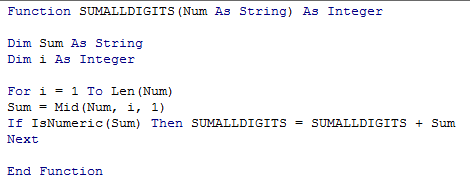Step 4: In B2 enter =sum to load all functions contain SUM. Verify that SUMALLDIGITS is displayed.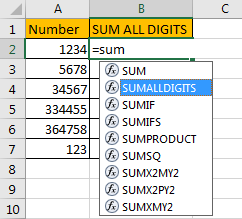Step 5: Select SUMALLDIGITS function, select A1, then click Enter. Verify that all digits’ total is calculated correctly.